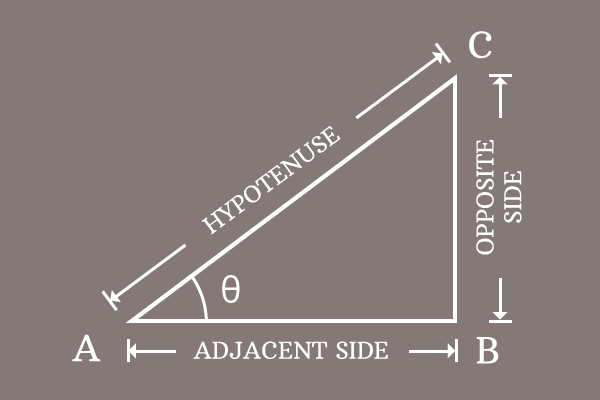# Quotient identity of Cosine and Sine with Cotangent

The quotient relation of cosine and sine functions with cotangent function is called the quotient identity of cosine and sine functions with cotangent function.

## Formula

$\dfrac{\cos{\theta}}{\sin{\theta}} \,=\, \cot{\theta}$

### Introduction

The trigonometric function cosine can be divided by sine function and the quotient of them represents another trigonometric function cotangent. The quotient relation of cosine and sine functions with cotangent function is used as a trigonometric formula in mathematics.

#### Proof

$\Delta BAC$ is a right angled triangle and the angle of the triangle is taken as theta ($\theta$).##### Define Sine and Cosine functions

Write sine and cosine functions in terms of ratio of the sides for the right angled triangle $BAC$.

$\sin{\theta} \,=\, \dfrac{Length \, of \, Opposite \, side}{Length \, of \, Hypotenuse} \,=\, \dfrac{BC}{AC}$

$\cos{\theta} \,=\, \dfrac{Length \, of \, Adjacent \, side}{Length \, of \, Hypotenuse} \,=\, \dfrac{AB}{AC}$

##### Divide Cosine function by Sine

Divide Cosine function by the sine function and then simplify it to get the quotient of them.

$\dfrac{\cos{\theta}}{\sin{\theta}} \,=\, \dfrac{\dfrac{AB}{AC}}{\dfrac{BC}{AC}}$

$\implies$ $\dfrac{\cos{\theta}}{\sin{\theta}}$ $\,=\,$ $\dfrac{AB}{AC} \times \dfrac{AC}{BC}$

$\implies$ $\dfrac{\cos{\theta}}{\sin{\theta}}$ $\,=\,$ $\dfrac{AB}{BC} \times \dfrac{AC}{AC}$

$\implies$ $\dfrac{\cos{\theta}}{\sin{\theta}}$ $\,=\,$ $\dfrac{AB}{BC} \times \require{cancel} \dfrac{\cancel{AC}}{\cancel{AC}}$

$\implies$ $\dfrac{\cos{\theta}}{\sin{\theta}}$ $\,=\,$ $\dfrac{AB}{BC} \times 1$

$\implies$ $\dfrac{\cos{\theta}}{\sin{\theta}}$ $\,=\,$ $\dfrac{AB}{BC}$

##### Expressing quotient of cosine by sine functions

The quotient of cosine function by sine function is a ratio of two sides. In fact, the ratio of length of the adjacent side ($AB$) by length of opposite side ($BC$) represents cotangent function according to the principle definition of the cotangent function.

$\cot{\theta} \,=\, \dfrac{Length \, of \, Adjacent \, side}{Length \, of \, Opposite \, side}$

$\implies \cot{\theta} \,=\, \dfrac{AB}{BC}$

Therefore, it has proved that the quotient of cosine function by sine function is equal to the cotangent function mathematically.

$\,\,\, \therefore \,\,\,\,\,\, \dfrac{\cos{\theta}}{\sin{\theta}} \,=\, \cot{\theta}$

Email subscription
Math Doubts is a best place to learn mathematics and from basics to advanced scientific level for students, teachers and researchers. Know more
Follow us on Social Media
###### Math Problems

Learn how to solve easy to difficult mathematics problems of all topics in various methods with step by step process and also maths questions for practising.

Learn more